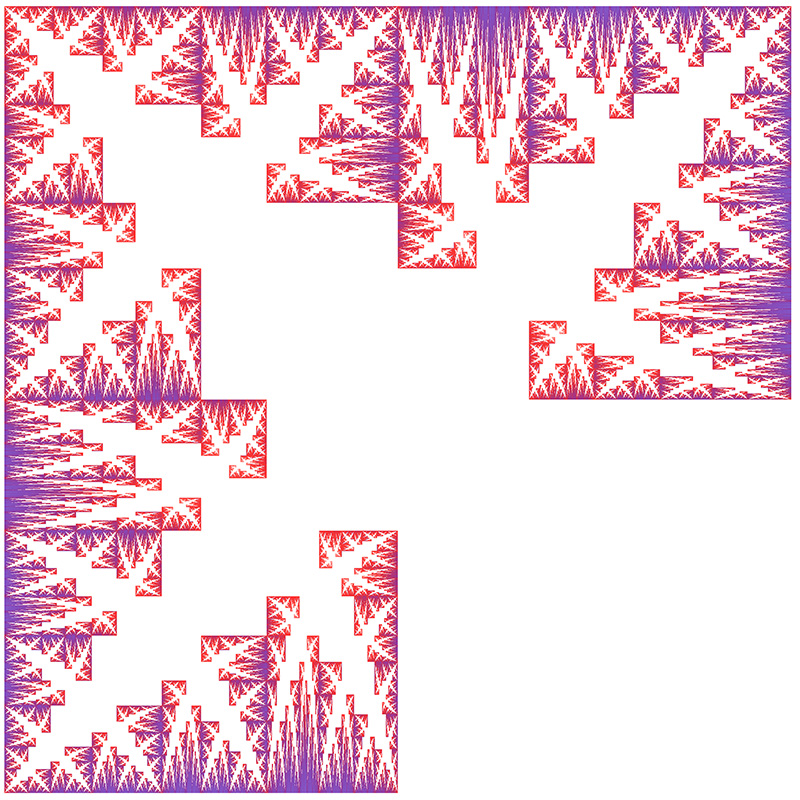# Inverse Tree

Created by Paul Bourke
Contributed by Roger Bagula

This is a classic IFS series (xn+1,yn+1) = f(xn,yn).

f(x,y) is chosen from one of the following with equal probability.
f(x,y) = (x/2 , y/2)
f(x,y) = (1 / (x+1) , y / (y+1))
f(x,y) = (x / (x+1) , 1 / (y+1))
The range of f(x,y) or 0 to 1 on both axes.